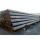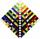# Cinema

Cinema auditorium is built for 3300 people. The first row is planned for 36 seats and each next gradually 4 more. How many rows of seats will have auditorium?

Result

n =  33

#### Solution:Checkout calculation with our calculator of quadratic equations.

Leave us a comment of example and its solution (i.e. if it is still somewhat unclear...):

Showing 1 comment:Sion
I like this question#### To solve this example are needed these knowledge from mathematics:

Looking for help with calculating roots of a quadratic equation? Do you have a linear equation or system of equations and looking for its solution? Or do you have quadratic equation?

## Next similar examples:

1. Cinema 4In cinema are 1656 seats and in the last row are 105 seats , in each next row 3 seats less. How many are the total rows in cinema?
2. SeatsSeats in the sport hall are organized so that each subsequent row has five more seats. First has 10 seats. How many seats are: a) in the eighth row b) in the eighteenth row
3. Sum of membersWhat is the sum of the first two members of the aritmetic progression if d = -4.3 and a3 = 7.5?
4. Sequence 2Write the first 5 members of an arithmetic sequence a11=-14, d=-1
5. Sequence 3Write the first 5 members of an arithmetic sequence: a4=-35, a11=-105.
6. SequenceWrite the first 7 members of an arithmetic sequence: a1=-3, d=6.
7. AP - simpleDetermine the first nine elements of sequence if a10 = -1 and d = 4
8. SequenceWrite the first 6 members of these sequence: a1 = 5 a2 = 7 an+2 = an+1 +2 an
9. TubesIron tubes in the warehouse are stored in layers so that each tube top layer fit into the gaps of the lower layer. How many layers are needed to deposit 100 tubes if top layer has 9 tubes? How many tubes are in bottom layer of tubes?
10. AS sequenceIn an arithmetic sequence is given the difference d = -3 and a71 = 455. a) Determine the value of a62 b) Determine the sum of 71 members.
11. EquationEquation ? has one root x1 = 8. Determine the coefficient b and the second root x2.
12. Theorem proveWe want to prove the sentence: If the natural number n is divisible by six, then n is divisible by three. From what assumption we started?
13. DiscriminantDetermine the discriminant of the equation: ?
14. SeriesYour task is express the sum of the following arithmetic series for n = 14: S(n) = 11 + 13 + 15 + 17 + ... + 2n+9 + 2n+11
15. RootsDetermine the quadratic equation absolute coefficient q, that the equation has a real double root and the root x calculate: ?
16. Solve 3Solve quadratic equation: (6n+1) (4n-1) = 3n2
17. SequenceBetween numbers 1 and 53 insert n members of the arithmetic sequence that its sum is 702.